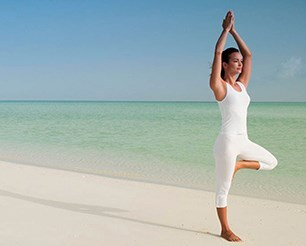# Spectacular Summer

A healthy mind is the key factor for a healthy body…wellness must include a happy mind.

- His Holiness the Dalai Lama,
2016 Global Wellness Summit

At this time of year, the countdown to the summer surf is underway but the collective energy of people is starting to wane, tempers are short, nerves are frayed and there is limited capacity for new ideas or routines. While we focus on getting the beach body ready, we tend to forget to turn inward to our mind to reflect on the year that has been and where we want to go.

Yoga gives you that space to 'work-in' as well as 'work-out...

Be sure to make YOU a priority by bringing yourself to your mat.
Stay up do date with our social media links below:

 table div table+table+table+table div table{width:100%;padding:0}table div table+table+table+table div table table{padding:0;float:left!important;width:26.315%!important}table div table+table+table+table div table td{padding-left:75px;padding-right:75px}table div table+table+table+table div table table+table,table div table+table+table+table div table table+table+table{float:left!important;width:26.315%!important}table div table+table+table+table div table table td,table div table+table+table+table div table table+table td,table div table+table+table+table div table table+table+table td{padding-left:0;padding-right:20px}table div table+table+table+table div table table+table+table+table{float:left!important;width:21.054999999999993%!important}table div table+table+table+table div table table+table+table+table td{padding-left:0;padding-right:0}/* styles */
 table div table+table+table+table+table div table{width:100%;padding:0}table div table+table+table+table+table div table img{width:96.23%;padding:0;float:none}table div table+table+table+table+table div table td{width:100%;padding:0 1.88% 18px}/* styles */## Holiday line-up

Reduced schedule from Saturday 16th December

LAST CLASS of the Year: Saturday 23rd December at 9 a.m. with Tarin

Studio re-opens Tuesday January 2nd 2018!

 table div table+table+table+table+table+table+table div table{width:100%;padding:0}table div table+table+table+table+table+table+table div table img{width:96.23%;padding:0;float:none}table div table+table+table+table+table+table+table div table td{width:100%;padding:0 1.88% 18px}/* styles */## Have you got the Yoga Warrior App?

 /* styles */ Click on the buttons below to download our very own app - Book your classes and stay up-to-date with class schedules and events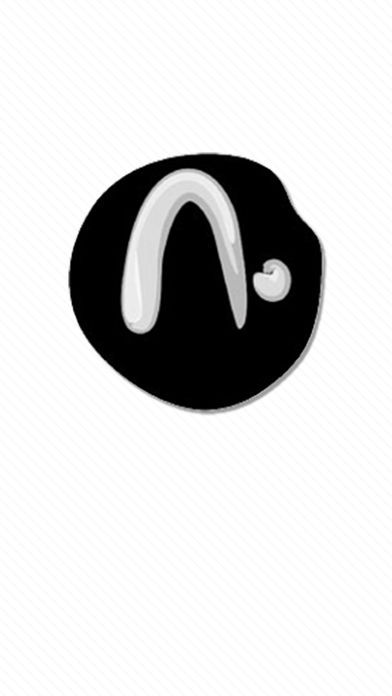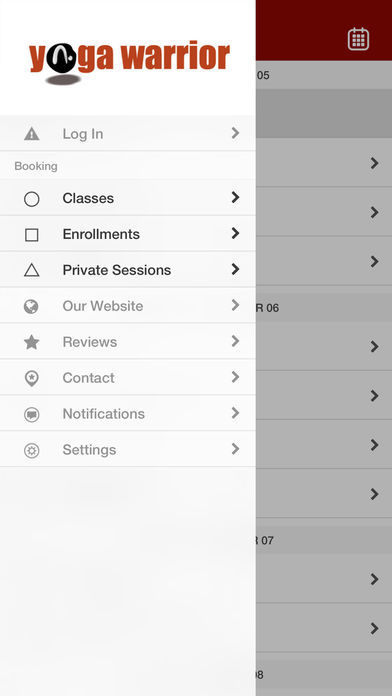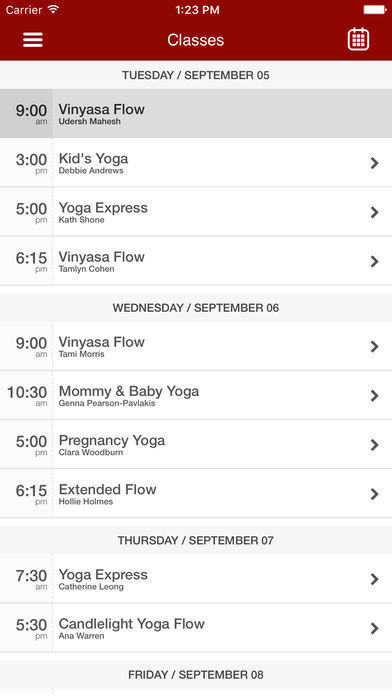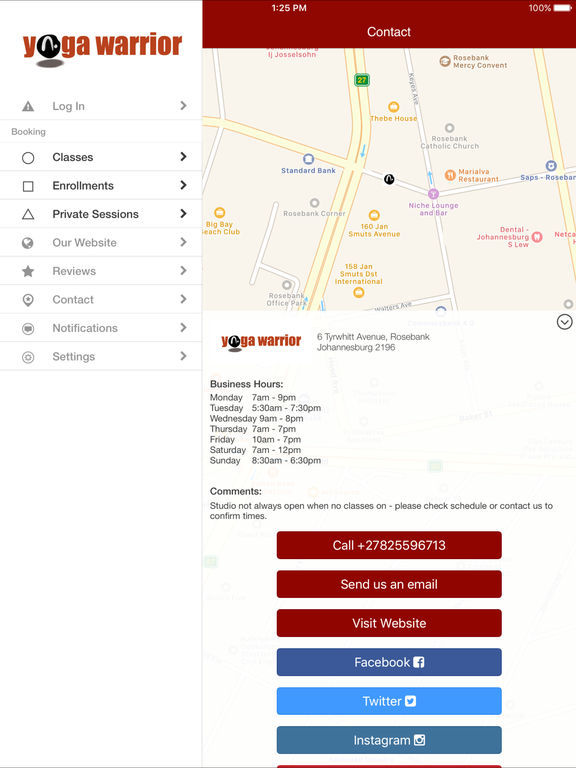table div table+table+table+table+table+table+table+table+table+table+table+table+table div table{width:100%;padding:0}table div table+table+table+table+table+table+table+table+table+table+table+table+table div table img{width:96.23%;padding:0;float:none}table div table+table+table+table+table+table+table+table+table+table+table+table+table div table td{width:100%;padding:0 1.88% 18px}/* styles */## Gift vouchers

Want to give your yogi friends / family a gift that keeps on giving?
We have plenty of options for gift vouchers valid all year round:
* Introductory special - R250 for 10 days
* Beginners Series voucher - R600 - next course starts January 14th/15th
* 5 or 10 class cards - from R475 to R1100
* Monthly unlimited pass - R850
* Private lessons - from R500
* etc., etc

## Year-end super special

Sign up NOW for 6-months unlimited yoga from Jan 2018 for only R750 per month on debit order and get rest of this year FREE!!!

Offer valid until 31st December 2017.

## Share the love - half price yoga for your friend

Do you have someone who you'd like to introduce to yoga?
Bring them along next time you come and they pay HALF PRICE!

Offer valid until 31st December 2017.

Check our website or email us for more info on price options.

 table div table+table+table+table+table+table+table+table+table+table+table+table+table+table+table+table div table{width:100%;padding:0}table div table+table+table+table+table+table+table+table+table+table+table+table+table+table+table+table div table img{width:96.23%;padding:0;float:none}table div table+table+table+table+table+table+table+table+table+table+table+table+table+table+table+table div table td{width:100%;padding:0 1.88% 18px}/* styles */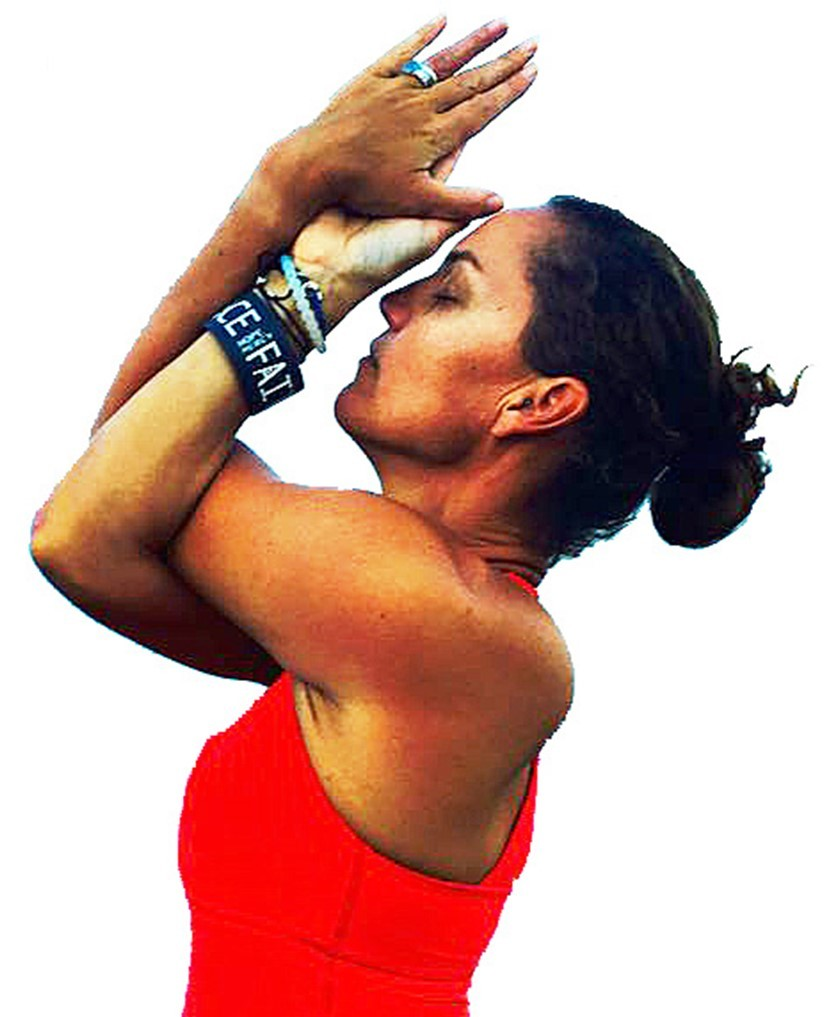## 2018 Teacher Training

As one of only six Registered Yoga Schools (RYS-200) in Johannesburg, Yoga Warrior is starting its next internationally accredited and well-known 200-hour teacher training on revised date of January 12th 2018 to give you more time to sign up... Cost: R24,950.
The course is structured over 6 months involving 8 weekends of tuition.

Course director Clara is an experienced Yoga teacher (E-YRT-200) with international training and experience who has developed and fine-tuned this life-altering course with key guest teachers over the past 11 years, training a number of Joburg's top teachers who now teach across town and beyond.

If you have a regular personal practice that you would like to take deeper or wish to share the beauty of yoga by becoming a teacher yourself and learning from one of Joburg's best teachers, drop us an email for more information or come and visit us for a class.

>>EARLY BIRD special price of R22,500 ends 30th November <<

 table div table+table+table+table+table+table+table+table+table+table+table+table+table+table+table+table+table+table div table{width:100%;padding:0}table div table+table+table+table+table+table+table+table+table+table+table+table+table+table+table+table+table+table div table img{width:96.23%;padding:0;float:none}table div table+table+table+table+table+table+table+table+table+table+table+table+table+table+table+table+table+table div table td{width:100%;padding:0 1.88% 18px}/* styles */## Mommy Yoga

Wednesdays are Mommy Yoga days!

Pregnancy Yoga classes taught by internationally trained pre-natal yoga teacher Clara are for expectant moms from 14 weeks and are held on Wednesdays at 5 p.m. for 70 mins.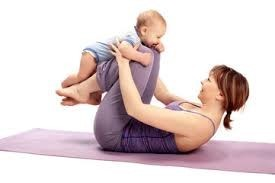Mommy & Baby Yoga classes for new moms and babies (from 6 weeks) taught by Genna are held on Wednesdays at 10:30 a.m.

 /* styles */ R450 per month on debit order for duration of your pregnancy and / or until baby is crawling (minimum contract 3 months). R650 for 5 classes valid for 2 months R150 for single class Email for more info.
 table div table+table+table+table+table+table+table+table+table+table+table+table+table+table+table+table+table+table+table+table+table+table div table{width:100%;padding:0}table div table+table+table+table+table+table+table+table+table+table+table+table+table+table+table+table+table+table+table+table+table+table div table img{width:96.23%;padding:0;float:none}table div table+table+table+table+table+table+table+table+table+table+table+table+table+table+table+table+table+table+table+table+table+table div table td{width:100%;padding:0 1.88% 18px}/* styles */## New Year, New You...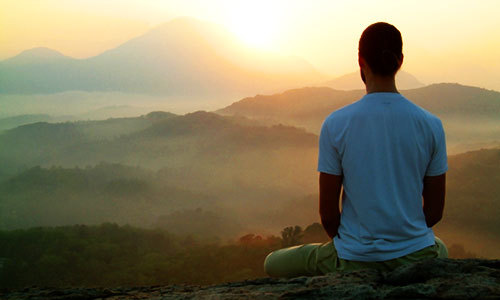## JANUARY 2018 BEGINNER YOGA SERIES

Kick off the new year with a commitment to your healthy self.....
OR if a friend or family member is looking to start afresh, get them to sign up to our First Beginner Series of 2018...
The 5 week series introduced the basics and fundamentals of yoga.

Next Series starts Sunday 14th & Monday 15th January, 2018.

The series includes 5 x 90 minute classes for only R600.

Bring a friend or partner and get R100 off! Couples pay R1100

Special price of R500 for repeat attendees scholars and students

Read more about it here.
Places fill up fast in the new year so be sure to sign up ASAP!

 table div table+table+table+table+table+table+table+table+table+table+table+table+table+table+table+table+table+table+table+table+table+table+table+table+table+table div table{width:100%;padding:0}table div table+table+table+table+table+table+table+table+table+table+table+table+table+table+table+table+table+table+table+table+table+table+table+table+table+table div table img{width:96.23%;padding:0;float:none}table div table+table+table+table+table+table+table+table+table+table+table+table+table+table+table+table+table+table+table+table+table+table+table+table+table+table div table td{width:100%;padding:0 1.88% 18px}/* styles */Yoga Warrior proudly supports the Sandton SPCA.
All donations for mat useage are matched by the studio and your contributions go towards feeding some needy animals.... We contribute ~R150 every month.

 table.module-27{width:62.83%;padding:0}table div table+table+table+table+table+table+table+table+table+table+table+table+table+table+table+table+table+table+table+table+table+table+table+table+table+table+table+table div table{width:62.83%;float:none;margin-left:auto;margin-right:auto;padding:0}table div table+table+table+table+table+table+table+table+table+table+table+table+table+table+table+table+table+table+table+table+table+table+table+table+table+table+table+table div table a{border:0 none;text-decoration:none}table div table+table+table+table+table+table+table+table+table+table+table+table+table+table+table+table+table+table+table+table+table+table+table+table+table+table+table+table div table img{width:100%!important;border:0 none;text-decoration:none}table div table+table+table+table+table+table+table+table+table+table+table+table+table+table+table+table+table+table+table+table+table+table+table+table+table+table+table+table div table td{width:100%;padding:0}/* styles */
 table div table+table+table+table+table+table+table+table+table+table+table+table+table+table+table+table+table+table+table+table+table+table+table+table+table+table+table+table+table div table{width:100%;padding:0}table div table+table+table+table+table+table+table+table+table+table+table+table+table+table+table+table+table+table+table+table+table+table+table+table+table+table+table+table+table div table img{width:96.23%;padding:0;float:none}table div table+table+table+table+table+table+table+table+table+table+table+table+table+table+table+table+table+table+table+table+table+table+table+table+table+table+table+table+table div table td{width:100%;padding:0 1.88% 18px}/* styles */# See you on the mat!

 Like   Tweet   Pin   in